## How to Convert Square Miles to Square Yards | Area Units

The image below represents square miles to square yards.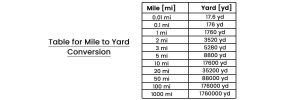To convert square miles to square yards, one essential parameter is needed and this parameter is Unit Value.

The formula for converting square miles to square yards:

1 Square Mile = 3097600 Square Yards

Let’s solve an example;
Find the conversion of square miles to square yards when the unit value is 30.

1 Square Mile = 3097600 Square Yards

Then,

30 Square Miles = (30 x 3097600) Square Yards
30 Square Miles = 92928000 Square Yards

Therefore, the square yard(s) is 92928000.

## How to Convert Acres to Square Yards | Area Units

The image below represents acres to square yards.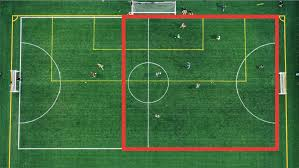To convert acres to square yards, one essential parameter is needed and this parameter is Unit Value.

The formula for converting acres to square yards:

1 Acre = 4840 Square Yards

Let’s solve an example;
Find the conversion of acres to square yards when the unit value is 10.

1 Acre = 4840 Square Yards

Then,

10 Acres = (10 x 4840) Square Yards
10 Acres = 48400 Square Yards

Therefore, the square yard(s) is 48400.

## How to Convert Square Yards to Square Miles | Area Units

The image below represents square yards to square miles.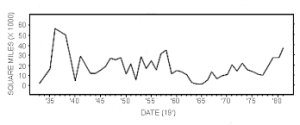To convert square yards to square miles, one essential parameter is needed and this parameter is Unit Value.

The formula for converting square yards to square miles:

1 Square Yard = 0.00000032283058 Square Miles

Let’s solve an example;
Find the conversion of square yards to square miles when the unit value is 4.

1 Square Yard = 0.00000032283058 Square Miles

Then,

4 Square Yards = (4 x 0.00000032283058) Square Miles
4 Square Yards = 0.00000129132232 Square Miles

Therefore, the square mile(s) is 0.00000129.

## How to Square Yards to Acres | Area Units

The image below represents square yards to acres.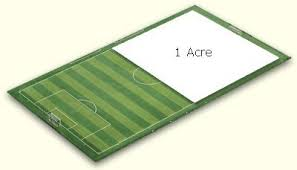To convert square yards to acres, one essential parameter is needed and this parameter is Unit Value.

The formula for converting square yards to acres:

1 Square Yard = 0.000207 Acres

Let’s solve an example;
Find the conversion of square yards to acres when the unit value is 7.

1 Square Yard = 0.000207 Acres

Then,

7 Square Yards = (7 x 0.000207) Acres
7 Square Yards = 0.001449 Acres

Therefore, the acre(s) is 0.001449.

## How to Convert Square Yards to Square Feet | Area Units

The image below represents square yards to square feet.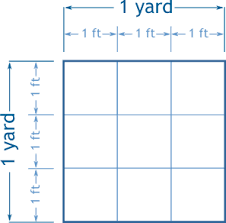To convert square yards to square feet, one essential parameter is needed and this parameter is Unit Value.

The formula for converting square yards to square feet:

1 Square Yard = 9 Square Feet

Let’s solve an example;
Find the conversion of square yards to square feet when the unit value is 12.

1 Square Yard = 9 Square Feet

Then,

12 Square Yards = (12 x 9) Square Feet
12 Square Yards = 108 Square Feet

Therefore, the square feet is 108.

## How to Convert Square Yards to Square Inches | Area Units

The image below represents square yards to square inches.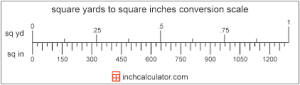To convert square yards to square inches, one essential parameter is needed and this parameter is Unit Value.

The formula for converting square yards to square inches:

1 Square Yard = 1296 Square Inches

Let’s solve an example;
Find the conversion of square yards to square inches when the unit value is 3.

1 Square Yard = 1296 Square Inches

Then,

3 Square Yards = (3 x 1296) Square Inches
3 Square Yards = 3888 Square Inches

Therefore, the square inch(es) is 3888.

## How to Convert Square Yards to Square Kilometers | Area Units

The image below represents square yards to square kilometers.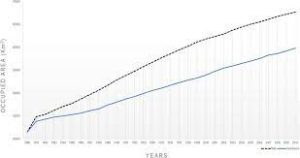To convert square yards to square kilometers, one essential parameter is needed and this parameter is Unit Value.

The formula for converting square yards to square kilometers:

1 Square Yard = 0.00000083612736 Square Kilometers

Let’s solve an example;
Find the conversion of square yards to square kilometers when the unit value is 14.

1 Square Yard = 0.00000083612736 Square Kilometers

Then,

14 Square Yards = (14 x 0.00000083612736) Square Kilometers
14 Square Yards = 0.00001170578304 Square Kilometers

Therefore, the square kilometer(s) is 0.0000117.

## How to Convert Square Yards to Hectares | Area Units

The image below represents square yards to hectares.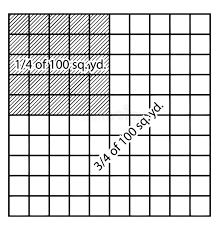To convert square yards to hectares, one essential parameter is needed and this parameter is Unit Value.

The formula for converting square yards to hectares:

1 Square Yard = 0.000084 Hectares

Let’s solve an example;
Find the conversion of square yards to hectares when the unit value is 2.

1 Square Yard = 0.000084 Hectares

Then,

2 Square Yards = (2 x 0.000084) Hectares
2 Square Yards = 0.000168 Hectares

Therefore, the hectare(s) is 0.000168.

## How to Convert Square Yards to Square Meters | Area Units

The image below represents square yards to square meters.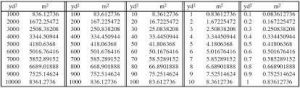To convert square yards to square meters, one essential parameter is needed and this parameter is Unit Value.

The formula for converting square yards to square meters:

1 Square Yard = 0.836127 Square Meters

Let’s solve an example;
Find the conversion of square yards to square meters when the unit value is 4.

1 Square Yard = 0.836127 Square Meters

Then,

4 Square Yards = (4 x 0.836127) Square Meters
4 Square Yards = 3.344508 Square Meters

Therefore, the square meter(s) is 3.344508.

## How to Convert Square Yards to Square Centimeters | Area Units

The image below represents square yards to square centimeters.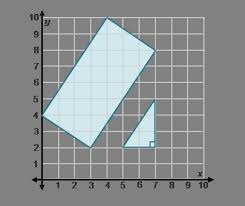To convert square yards to square centimeters, one essential parameter is needed and this parameter is Unit Value.

The formula for converting square yards to square centimeters:

1 Square Yard = 8361.274 Square Centimeters

Let’s solve an example;
Find the conversion of square yards to square centimeters when the unit value is 6.

1 Square Yard = 8361.274 Square Centimeters

Then,

6 Square Yards = (6 x 8361.274) Square Centimeters
6 Square Yards = 50167.644 Square Centimeters

Therefore, the square centimeter(s) is 50167.64